# General Wallpaper Probability Problem .pdf

### File information

Original filename: General Wallpaper Probability Problem.pdf
Author: Ryan

This PDF 1.5 document has been generated by MicrosoftÂ® Word 2016, and has been sent on pdf-archive.com on 13/03/2017 at 01:48, from IP address 76.173.x.x. The current document download page has been viewed 322 times.
File size: 694 KB (5 pages).
Privacy: public file

General Wallpaper Probability Problem.pdf (PDF, 694 KB)

### Document preview

WALLPAPER PROBABILITY
Problem Statement:
I have 274 unique wallpaper images stored on my phone. Every so often, 1 of the 274
wallpapers is chosen at random to be displayed on my home screen. What is the
expected number of wallpaper changes when the first duplication occurs?

Hand Calculations and Solution Development

Probability Mass Function Development
274
0
)(
)
274 274
274
1
𝑃𝑋 (2) = (
)(
)
274 274
274 273
2
𝑃𝑋 (3) = (
)(
)(
)
274 274 274
274 273 272
3
𝑃𝑋 (4) = (
)(
)(
)(
)
274 274 274 274
𝑃𝑋 (1) = (

.
.
.

𝑘

𝑃𝑋 (𝑘) = [∏
𝑖=2

276 − 𝑖 𝑘 − 1
](
)
274
274

; 1 ≤ 𝑘 ≤ 274

Expected Value Function
274

𝐸 [𝑋] = ∑ 𝑘 · 𝑃𝑋 (𝑘)
𝑘=1

Final Solution
274

𝑘

𝐸 [𝑋] = ∑ 𝑘 [[∏
𝑘=1

𝑖=2

276 − 𝑖 𝑘 − 1
](
)]
274
274

𝐸 [𝑋] ≈ 21.41891 is the expected number of wallpaper
changes when the first duplicate occurs.

MATLAB Verification
%Builds array of Probability for “the exact kth wallpaper is a repeat”
for k=1:274
x=1;
%Calculates Probability of (k-1) wallpapers being different
for n=2:k
x=x*((276-n)/274);
end
%Multiplies P(k-1 wallpapers being different) * P(kth wallpaper being a
%duplicate
y(k)=x*(k-1)/274;
end
%Calculates expected value using formula P(x)*x for all indices.
expected_value = 0;
cdf = 0;
for n=1:274
expected_value = expected_value + y(n)*n;
if (n-1)&gt;0
cdf(n) = cdf(n-1) + y(n);
end
end
expected_value
%Plots the probability mass function
a=1:1:274;
stem(a, y);
axis([0, 100, 0, 0.04]);grid
title('Probability Mass Function');
xlabel('Kth Wallpaper');
ylabel('Probability');
%Plots the cumulative distribution function
figure;
stairs(a, cdf);
hold;
axis([0, 100, 0, 1]);grid
title('Cumulative Distribution Function');
xlabel('Kth Wallpaper');
ylabel('Cumulative Probability');
_____________________________________________________________________________

MATLAB Output:
expected_value =
21.4189

Plotting for K &lt;= 100

C Programming Simulation
int run_count = 1000000000;
int random_number;
int total_wallpapers = 274;
int number_array;
int numbers_found;
int i;
int j;
int duplicate_found = 0;
double wallpapers_before_repeat = 0;
void Clear_Array()
{
int k;
for(k=0;k&lt;=275;k++)
{
number_array[k] = 0;
}
}
void main(void)
{
srand(time(NULL));
//Runs the trial "run_count" number of times
for(j=1;j&lt;=run_count;j++)
{
Clear_Array();
for(i=1;i&lt;=274;i++)
{
//Chooses a random wallpaper between 1 and 274
random_number = (rand() % total_wallpapers) + 1;
//Counts running total of wallpapers before a repeat
wallpapers_before_repeat += 1;
//Checks if wallpaper is a repeated wallpaper
if (number_array[random_number] == 1)
{
//Repeat is found - exits the loop and runs next trial
i=275;
}
//Checks if wallpaper is different
else
{
//Adds wallpaper to list of non-repeated wallpapers
number_array[random_number] = 1;
}
}
}
//Calculates E[X] and prints the result
printf("Results of E[X] after 1 billion iterations\n\
------------------------------------------\
\n\nE[X] = %lf\n\n", double(wallpapers_before_repeat/run_count));
}

Results of E[X] after 1 billion iterations
E[X] = 21.417616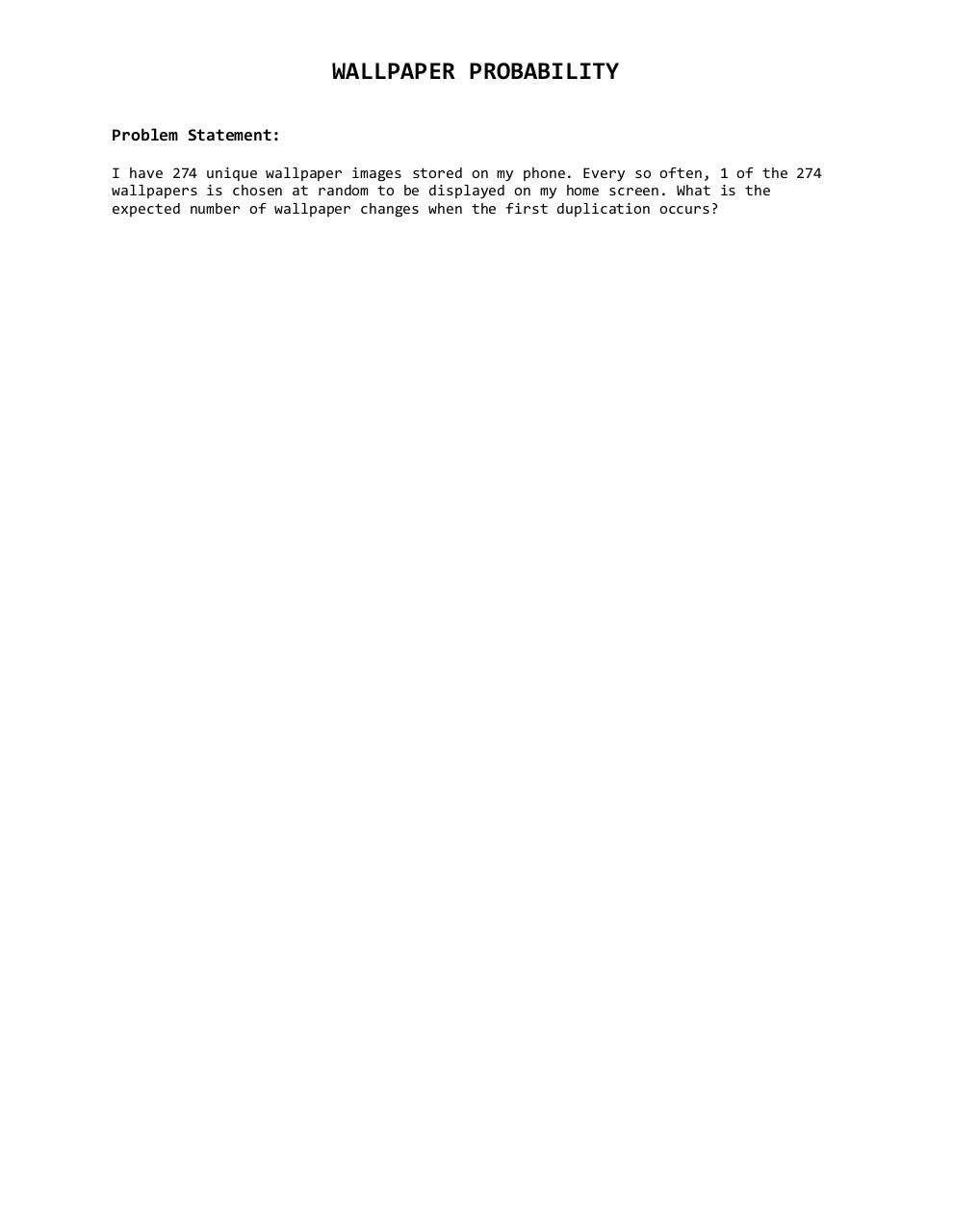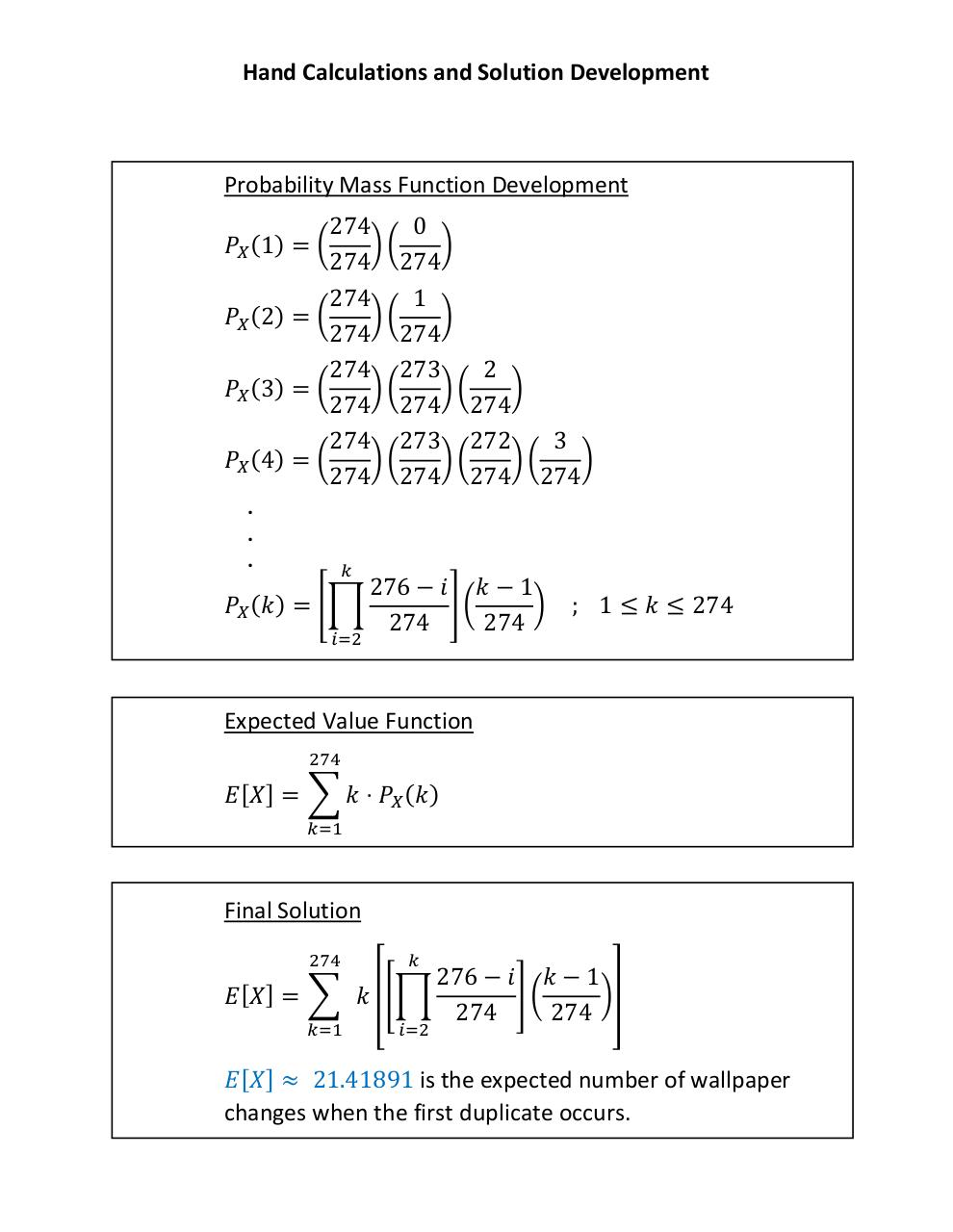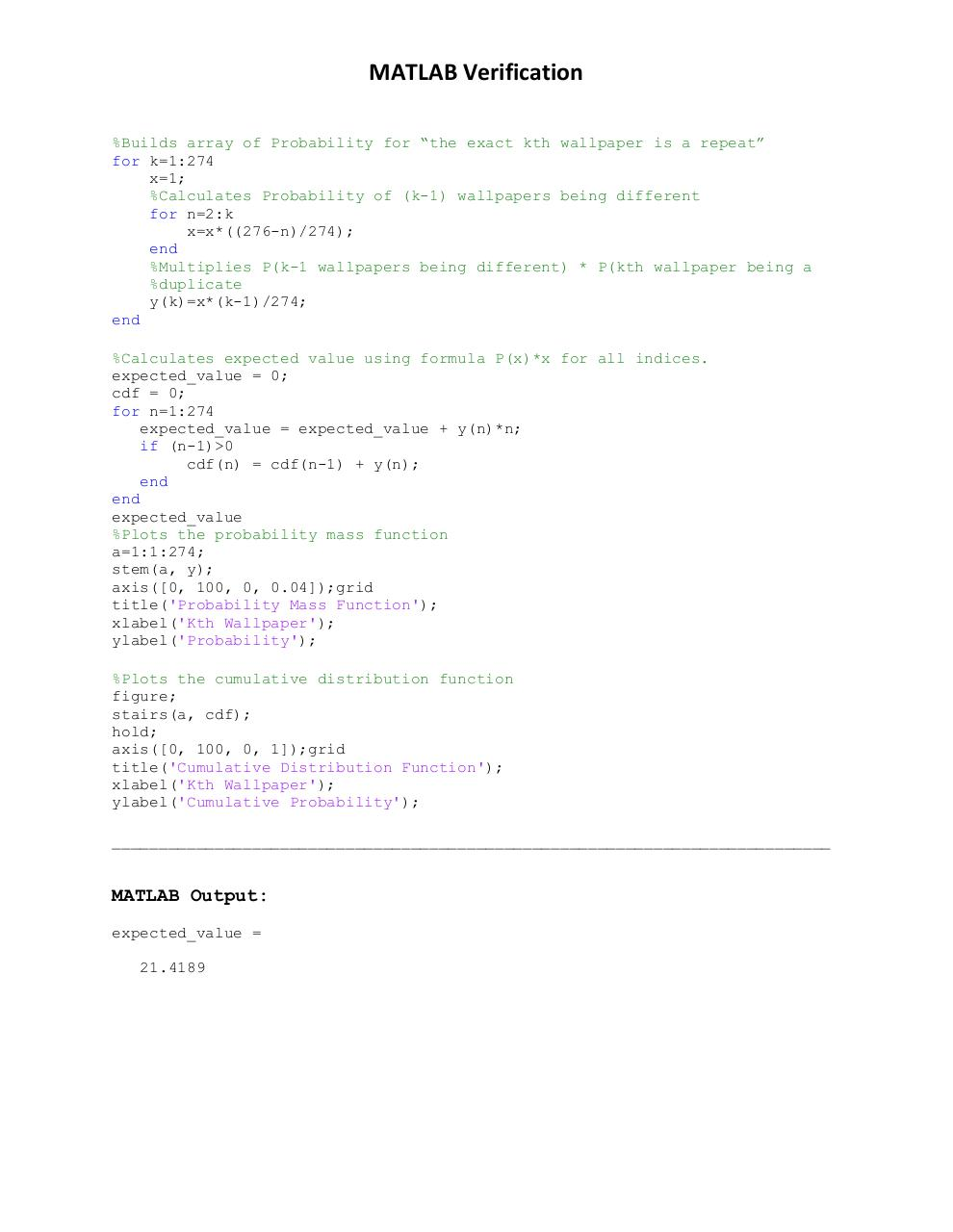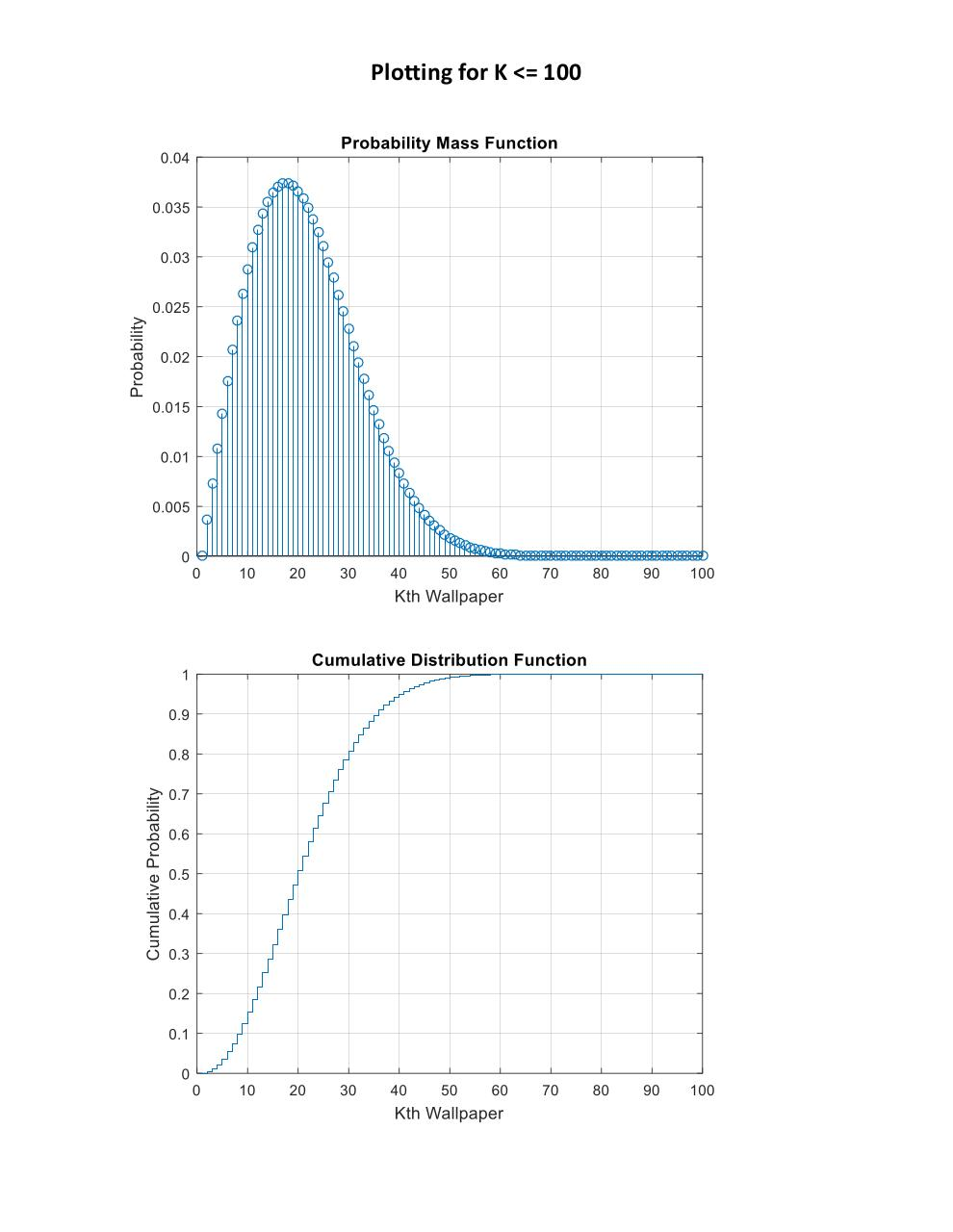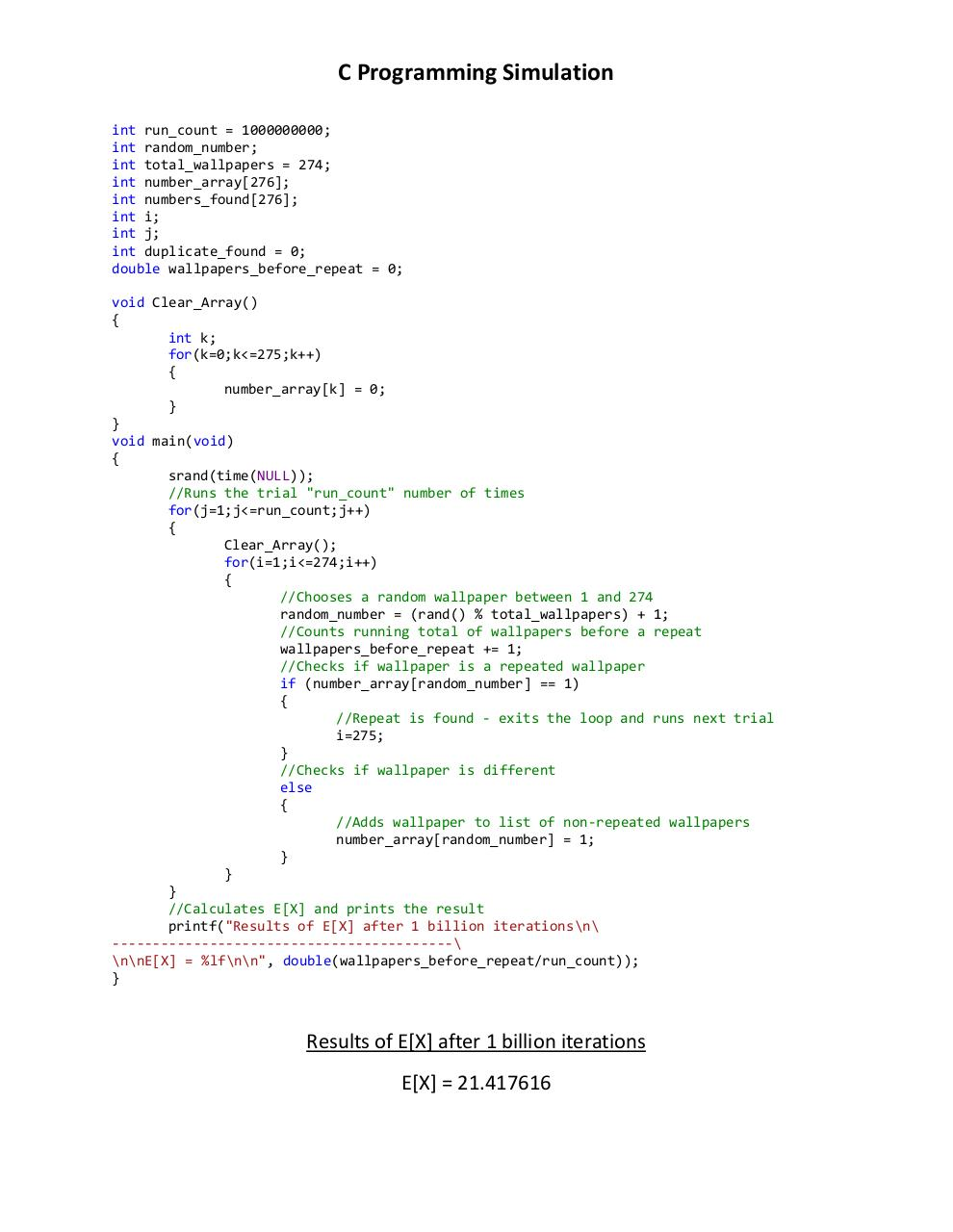#### HTML Code

Copy the following HTML code to share your document on a Website or Blog

#### QR Code### Related keywords